## Threshold Free Cluster Enhancement explained

#### The multiple comparison problem

In neuroimaging analysis one often is confronted with many electrodes/voxels and many timepoints, and often performs a statistical test on each of these electrode/timepoint combinations. This leads to a massive multiple comparison problem, as the probability to find a false positive is greatly enhanced. In the following example we assume independence of all data points . For instance with only 10 electrodes/voxels and 10 timepoints and an alpha of 0.05, the probability for a false positive is:

$$p(significance^*|H_0) = 1-(1-0.05)^{10*10}=99.4$$

*significance of at least one sample

But electrodes/voxels and timepoints usually are not independent. Contrary, data is rather smooth over electrodes, voxels and time. Therefore, by combining data points close in space (electrodes / voxels) and time using so-called cluster tests, one can try to partially circumvent this problem.

For the IICCSSS Summer School I made some slides explaining multiple comparison correction (link to slides, pptx), including Threshold Free Cluster Enhancement (TFCE). I explained cluster permutation tests in an earlier blog post, which you might want to read before this one.

#### Why TFCE and not cluster permutation tests?

In order to use cluster permutation tests, one typically first calculates some kind of statistics, in our example student t-values for each datapoint over subjects (or trials for single subject analyses). The one has to specify a cluster-threshold which defines the clusters. This threshold might miss broad but “weak” clusters, and focus only on “strong” but peaky clusters.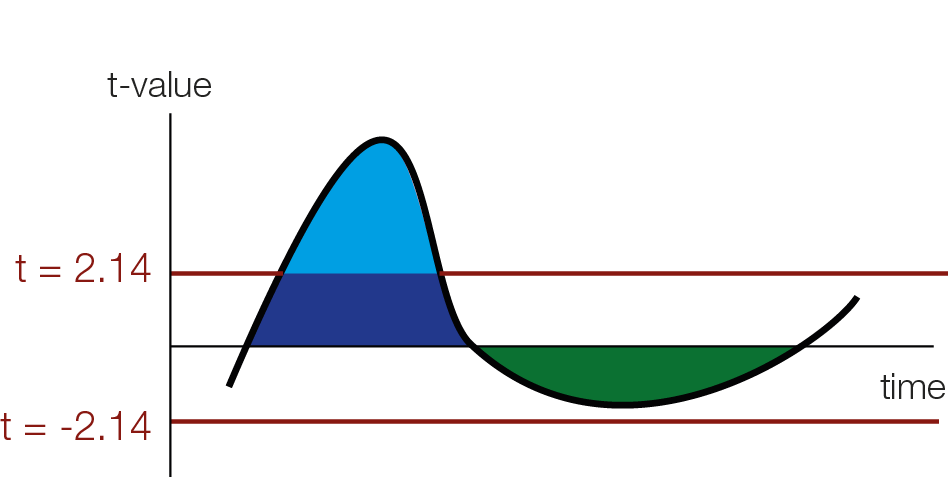As an example I depict students t-value of an effect over time. In this figure, the blue cluster will be detected (the thresholded cluster mass is depicted by the light blue area), but the broader green cluster would be missed

#### Threshold Free Cluster Enhancement

The intuition of TFCE is that we are going to try out all possible thresholds and see whether a given time-point belongs to a significant cluster under any of our set of cluster-thresholds. Instead of using cluster mass, we will use a weighted average between the cluster extend (e, how broad is the cluster, i.e. how many connected samples) and the cluster height (h, how high is the cluster, i.e. how large is the t-value / the evidence for an effect) according to the formula:

$$TFCE = \int_h e(h)^Eh^Hdh$$

for this blogpost, I will put the weights for the extend E and for the height H to 1 (therefore height ‘counts’ the same as width). The usual defaults are E=0.5 and H=2.Note that the weights E and H of the TFCE formula $e^E$ and $h^H$ are set to 1 and therefore ommitted in the gif. A non-gif version can be found in this powerpoint presentation: https://cloud.wirdreibei.de/s/GE6nwpRTrQdD76n (CC-By)

As you can see we use a discrete sum, approximating the integral from above. Another difference between TFCE and cluster permutations, is that you generate a TFCE value for each sample.

For instance a hypothetical t-value of 3 (red square in the above animation) is boosted by belonging to a cluster and might receive the TFCE value of 10. The resulting TFCE values can be thought of as a local scaling according to the “clusterdness” of a sample. Note that local minima and maxima stay at the same spot, this is different to a smoothing operation which could move the location of maxima and minima in time or space.

Because we calculated a TFCE value for each sample, we can also calculate a p-value for each sample. In order to get the p-values, we use the same trick wie used with cluster permutation test: the permutation part. We permute conditions (building the $H_0$), calculate the TFCE values for the permutated set, and take the max(TFCE) over all time points and electrodes/voxels. Our observed TFCE value then is either likely or unlikely given our empirical distribution of max TFCE values (under the $H_0$). But note that the interpretation is not that of a typical p-value at an electrodes/voxel!.

#### Interpretation of significant TFCE

I admit I made the following mistake before, it is a very convenient and easy mistake to make: As an example, let’s observe a significant sample e.g. at 100ms, using the TFCE procedure. This does not mean that the sample at 100ms shows a significant effect. It only means, that there exist at least one cluster-threshold (remember we tested all of them), where this sample belongs to a significant cluster. In other words, samples can be pushed to significance solely by being close to a “truely significant” cluster, without showing evidence by themselves to be significant.

I found this pretty confusing. But in practice it is important: Because we don’t know which samples make a cluster a significant one (all of them? half of them? only a single sample?) we cannot say much about the single sample, only about the cluster.

So, in practice what we do is that we look and report the p-values, but in addition make a descriptive statement on the cluster extend. For instance, you could argue that the t-values that you put in TFCE (or cluster permutation) are very much compatible with an effect from X ms to Y ms. Similar statements are also recommended on the fieldtrip site or in this recent paper by Jona Sassenhagen 2018.

Don’t write: “We found a significant cluster starting from 100ms to 200ms with a median effect of 5µV [3.5, 4.7µV].” or even “Conditions differed significantly from 100ms to 200ms (multiple comparison corrected)”.
Write: “We found a significant difference between conditions. The difference was driven by an effect from 100ms to 200ms with a median effect of 5µV [3.5, 4.7µV] .” or “We found a significant cluster, most compatible with an effect from 100ms to 200ms with a median effect of 5µV [3.5, 4.7µV] “.

Dont write: “At t=125ms the conditions differed significantly (TFCE correction for multiple comparisons) with a median effect of 5µV [3.5, 4.7µV] “
Write: “We found significant difference between conditions (TFCE correction for multiple comparisons). This difference was driven by a cluster starting at 125ms with a median effect of 5µV [3.5, 4.7µV] .”

These messages are much less snappy, sexy, short or easy to understand. The important bit is to signal to the reader that the cluster permutation test does not state significance about a single timepoint or electrode/voxel, but only indicates a significant difference somewhen/re between your conditions.

This problem has been recently discussed on twitter. One proposed alternative is All-Resolution-Inference. There is a barebone R-implementation in the hommel package and I would be interested in translating it to matlab to be readily usable with cluster permutation for EEG data.

Thanks for personal discussions (these are not endorsements, all mistakes in this blogpost are mine!) with Eelke Spaak, Robert Oostenveld, René Scheeringa, Olaf Dimigen & Phillip Alday + twitter interactions with Guillame A Rousselet, Cyril Pernet, Thomas Nichols and Martin Hebart. Thanks to Anna Lisa Gert for critical comments on this blogpost.

## Simulating EEG/ERP Data with SEREEGA & multiple comparison corrections

For the $i^2 c^2 s^3$ summer school I simulated quite a bit of data and analyzed them with several common multiple comparison methods. I used the SEREEGA toolbox for the simulation. All the MatLab-code can be found at the end of this post. In a follow-up blogpost I will extend the toolbox to continuous data that we can analyze with the unfold toolbox.

First, I simulated data based on three effects: Two early dipoles representing the P100, one right lateralized for the N170 and a deep one for the P300.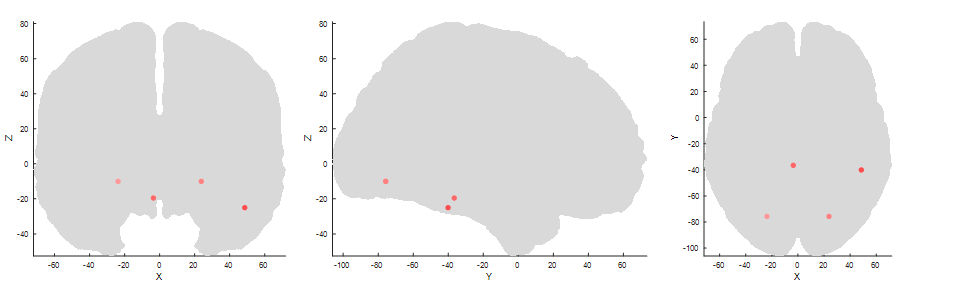Two symmetric sources for the P1 in the occipital cortex, one lateralized to the right cortex (third panel right most) for the N170 and a deep one, central for the P300.

I added brown and white noise to the simulated epochs. An exemplary eegplot shows that it kind of looks like EEG data.

I generate two conditions with two different condition-differences, one on the N170 and one on the P300 . The same data are depicted in the following three plots:

In the first row we see a butterfly plot of activation in all channels (the colored channels are depicted in the next plot). In the first topoplot-row we have the average activation and in the last row we have the condition differences.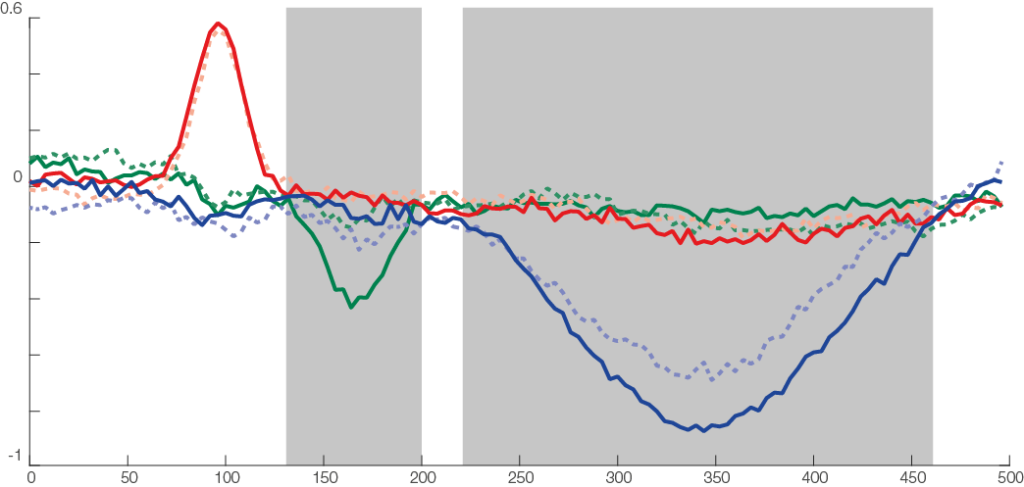The three types of components: a short but relatively strong (P100, red), a similar effect, short but strong (N170, condition difference) and a long effect (P300, with weak condition effect). Highlighted gray areas are approximate regions where the components showed condition differences. The noise was a mix between brown and white noise.

The red line is the P100, occipital effect. No difference between conditions. The green one is the N170, temporal effect. Only visible in one condition. The blue one is a P300 like, deep effect. Difference in amplitude between conditions.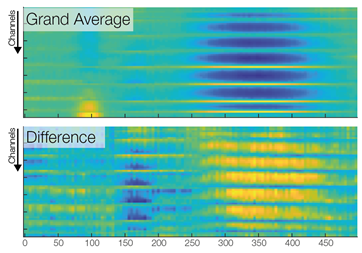A third way to look at the same data. Color indicates activation (blue => negative µV, yellow=> positive µV). Each row is one channel, each column one time point. Using such a plot, one looses spatial relations between the channels , but gains an nice overview of all the data. Note that the colorscales are different between the two panels.

Now we are ready to do some statistical testing and multiple comparison correction. I choose to compare uncorrected, bonferroni-holms, FDR (Benjamini-Hochberg), clustermass cluster permutation testing (LIMO toolbox) and threshold free cluster enhancement (EPT-TFCE toolbox).

In this instance, uncorrected p-value did not so bad, Bonferroni-Holms is, as expected, quite conservative. FDR hat troubles with the elongated cluster and TFCE/Cluster-permutation with the short one.

Note: This is only a single simulation. In order to move from these anectotal findings to proper statements, one would need to repeat the simulation 1000 times and see how often which samples were deemed significant (see Groppe 2011).

Thanks to Anna Lisa Gert for help with writing this post.

This is the code I used, it is a bit messy because it was programmed in a tutorial-style and I kept my debug-statements in there.

rng(1)
eeglab
end
% Threshold Free cluster enhancement
%% SEREEGA Data Generation
cfg = struct('debug',0)

if cfg.debug
end

%% set seed

%% noise
noise_brown = struct( ...
'type', 'noise', ...
'color', 'brown', ...
'amplitude', 2);
noise_brown = utl_check_class(noise_brown);

noise_white = struct( ...
'type', 'noise', ...
'color', 'white', ...
'amplitude', 2);
noise_white = utl_check_class(noise_white);

%% visual Cortex N170
vis = [];

vis.signal{1} = struct();
vis.signal{1}.peakLatency = 170;      % in ms, starting at the start of the epoch
vis.signal{1}.peakWidth = 100;        % in ms
vis.signal{1}.peakAmplitude = -5;      % in microvolt
vis.signal{1} = utl_check_class(vis.signal{1}, 'type', 'erp');
vis.signal{2} = noise_brown;

% right visual cortex
vis.source= lf_get_source_nearest(lf, [50 -40 -25]);
if cfg.debug
plot_source_location(vis.source, lf, 'mode', '3d','shrink',0);
end

% vis.orientation = utl_get_orientation_pseudotangential(vis.source,lf);
vis.orientationDv = [0 0 0]; % orientation does not change between trials
vis.orientation = [0.5,-0.46,1]; % I looked at the maps and found that this prientation most closely looked like a N170

if cfg.debug
%% This Code I used to generate random orientations and inspect the leadfield projections
for k = 1:10
vis.orientation = utl_get_orientation_random(1)
plot_source_projection(vis.source, lf, 'orientation', vis.orientation,'orientedonly',1);
%     title(vis.orientation)
end
end
%% Generate P100, take from SEREEGA Toolbox
p100 = utl_get_component_fromtemplate('visual_p100_erp',lf);
if cfg.debug
% Visualize the response
plot_signal_fromclass(vis.signal{1}, epochs);
end

%% Generate P300
p3 = [];

p3.signal{1} = struct();
p3.signal{1}.peakLatency = 350;      % in ms, starting at the start of the epoch
p3.signal{1}.peakWidth = 400;        % in ms
p3.signal{1}.peakAmplitude = -3;      % in microvolt
p3.signal{1} = utl_check_class(p3.signal{1}, 'type', 'erp');
p3.signal{2} = noise_brown;

% somewhere deep in the brain
p3.source= lf_get_source_nearest(lf, [0 -40 -25]);
if cfg.debug
%% visualize it using SEREEGA
plot_source_location(p3.source, lf, 'mode', '3d');
end

p3.orientation = utl_get_orientation_pseudotangential(p3.source,lf);
p3.orientationDv = [0 0 0];

p3.orientation = [0.03,-0.16,1];
if cfg.debug
%% again find an orientation that somehow matches P300topography
for k = 1:10
p3.orientation = utl_get_orientation_random(1)
plot_source_projection(p3.source, lf, 'orientation', p3.orientation,'orientedonly',1);
end
end

%% generate 25 random noise sources and combine all components
sources = lf_get_source_spaced(lf, 10, 25);
noise_brown.amplitude = 5;
comps = utl_create_component(sources, noise_brown, lf);
[comps1, comps2] = deal(comps);

% add P100 to noise components
comps1(end+1:end+2) = p100; %bilateral
comps2(end+1:end+2) = p100;

% N170 with condition difference
vis.signal{1}.peakAmplitude = -3;
comps1(end+1) = vis;
vis.signal{1}.peakAmplitude = -1;
comps2(end+1) = vis;

% broad, weak P300, with condition difference
p3.signal{1}.peakAmplitude = -10;
comps1(end+1) = p3;
p3.signal{1}.peakAmplitude = -12;
comps2(end+1) = p3;
%% Generate The EEG Data
epochs = struct();
epochs.n = 50;             % the number of epochs to simulate
epochs.srate = 250;        % their sampling rate in Hz
epochs.length = 500;

data1 = generate_scalpdata(comps1, lf, epochs);
data2 = generate_scalpdata(comps2, lf, epochs);
EEG1 = utl_create_eeglabdataset(data1, epochs, lf, 'marker', 'event1');
EEG2 = utl_create_eeglabdataset(data2, epochs, lf, 'marker', 'event2');
EEG = utl_reorder_eeglabdataset(pop_mergeset(EEG1, EEG2));

%% First inspection, this should match the intended topographies
pop_topoplot(EEG, 1, [0 100 170,300], '', [1 8]);
%% ERP-Like Figure
figure,
findtype = @(type)cellfun(@(x)strcmp(type,x),{EEG.event.type});

ploterp = @(chan)plot(EEG.times,...
[mean(squeeze(EEG.data(chan,:,findtype('event1'))),2),...
mean(squeeze(EEG.data(chan,:,findtype('event2'))),2)]);
figure,ploterp(44),title(EEG.chanlocs(44).labels)
hold on,ploterp(63),title(EEG.chanlocs(63).labels)
hold on,ploterp(30),title(EEG.chanlocs(30).labels)

%% Within-Subject T-Test using LIMO

[m,dfe,ci,sd,n,t,p] = limo_ttest(2,EEG.data(:,:,findtype('event1')),EEG.data(:,:,findtype('event2')),0.05);
%% simple imagesc plots
figure,subplot(2,1,1),imagesc(EEG.times,1:64,mean(EEG.data,3));box off
subplot(2,1,2),imagesc(EEG.times,1:64,m),box off
% Show data
figure
imagesc(m)

figure
imagesc(t)
%% Prepare statistics

%%
ept_tfce_nb = ept_ChN2(EEG.chanlocs,0); % the 1 to plot
tfce_res = ept_TFCE(...
permute(EEG.data(:,:,findtype('event1')),[3 1 2]),...
permute(EEG.data(:,:,findtype('event2')),[3,1,2]),EEG.chanlocs,'nperm',800,'flag_save',0,'ChN',ept_tfce_nb)

%%
t_h0 = nan([size(EEG.data,1),size(EEG.data,2),800]);
for b = 1:800
fprintf('%i/%i\n',b,800)
r_perm      = randperm(epochs.n*2); % Consider using Shuffle mex here (50-85% faster)...

nData       = EEG.data(:,:,r_perm);
sData{1}    = nData(:,:,1:epochs.n);
sData{2}    = nData(:,:,(epochs.n+1):(epochs.n*2));

t_h0(:,:,b) = (mean(sData{1},3)-mean(sData{2},3))./sqrt(var(sData{1},[],3)/epochs.n+var(sData{2},[],3)/epochs.n);

end
p_h0 = tpdf(t_h0,199);
%% Generate Cluster Permutation
limo_nb = limo_neighbourdist(EEG,50);
cluster_p(isnan(cluster_p(:))) = 1;
% F_tfce = limo_tfce(2,t.^2,limo_nb);

%% show significance methods
figure,
for k = 0:4
switch k
case 0
plot_p = p;
case 1
plot_p = bonf_holm(double(p),0.05);
case 2
[~,~,~,plot_p] = fdr_bh(p,0.05,'pdep','no'); % see groppe 2011 why pdep is fine

case 3
plot_p = cluster_p;

case 4
plot_p = tfce_res.P_Values;

end
plot_data= plot_p;
plot_data = log10(plot_data);
subplot(5,1,(k)+1)
eegvis_imagesc(m,t,'chanlocs',EEG.chanlocs,'times',EEG.times,...
%     caxis([-7,0])
%     if k ~=4
%         axis off
%     end
%     box off
box off
end

%%
hA = plot_topobutter(mean(EEG.data,3),EEG.times,EEG.chanlocs,'colormap',{'div'},'quality',40,'n_topos',12);

%%
figure
hA = plot_topobutter(cat(3,mean(EEG.data,3),m),EEG.times,EEG.chanlocs,'quality',32,'individualcolorscale','row','highlighted_channel',[44,63]);

plot_source_location([p100.source,vis.source,p3.source], lf, 'mode', '2d');



## Random Plot (constricting radial sinus)

This was the result of a (inconclusive) test of how the blind spot fills in things

theta = linspace(0, 2*pi, 50).';
KbName('UnifyKeyNames')
Factor = 10;
figure
while true

[X, Y] = meshgrid(linspace(-pi,pi,1000),linspace(-pi,pi,1000));
dist = sqrt((X.^2 + Y.^2));
imagesc(sin(dist*Factor))
colormap('gray'), axis equal
drawnow;
[~,keyCode] = KbWait;

kb = KbName(find(keyCode));
if ~iscell(kb)
switch kb
case 'LeftArrow'
Factor = 0.9*Factor;
case 'RightArrow'
Factor = 1.1*Factor;
case 'Esc'
continue
%         break
end
%                     display(kb)

end
end

## Thesis Art

I was a supervisor for Katharina Groß’s Bachelor’s Thesis. You can find the resulting paper (!) here on biorXiv

In her project we developed a new test battery to benchmark eye-trackers in many different tasks. We concurrently measured Pupil Labs Glasses and an Eyelink 1000. In the thesis art I selected six of the tasks and visualized the data of one subject using the letters of her thesis. The tasks I used are: Fixation Grid, Smooth Pursuit, Microsaccades, Blinks, Pupil Dilation and Free Viewing.

The idea of “thesis art” is to inspire discussion with persons who do not have an academic background or work in a different field. The thesis is hidden in the drawer, but the poster is out there at the wall for everyone to see. You can find all past thesis art pieces here

## No evidence for periodicity in reaction time histograms

### Introduction

In my last lab we discussed findings on the periodicity of reaction times (e.g. referred in Van Rullen 2003). These studies are usually old (Starting with Harter 1968, Pöppel 1968), with small N and not many trials. There was also a extensive discussion in the Max-Planck Journal “Naturwissenschaften” in the 90s (mostly in German, e.g. Vorberg & Schwarz 1987). A methodological critique is from Vorberg & Schwarz 1987. More discussion in Gregson (Gregson, Vorberg, Schwarz 1988). A new method to analyse periodicity is proposed by Jokeit 1990.

This is the newest research I could find on this topic.

### Analysing a large corpus of RT-data

I stumbled upon a large reaction time dataset (816 subjects, á 3370 trials, 2.3 million RTs)  from the English Lexicon Project (Balota et al 2007) and decided to look for these oscillations in reaction times .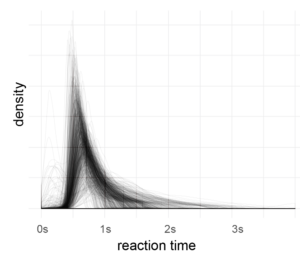After outlier correction (3*mad rule, see below), I applied a fourier-transformation on the histogram (1ms bins, accuracy of RT=1ms). Then I looked for peaks in the spectrum which are consistent over subjects.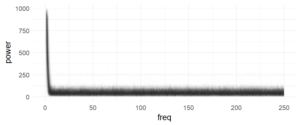Each subject is one line, no effect is visible here (in a log-scaled y-axis also no effect can be seen). The range (above ~7Hz) of the subject variance is roughly between +0 and 100

The following graph summarizes  the above graph (blue-smoother-curve = loess, span=0.1, each dot = mean over 800 subjects)):There are no peaks in the spectrum which I consider as consistent over subjects. I included higher frequencies (up to 250Hz) to get a visual estimate of the noise level (at such high frequencies, an effect seems utterly implausible). But of course, I’m ignoring  within subject information (i.e. a mixed model of some sort could be appropriate).

### Conclusions

In this large dataset, I cannot find periodicities of reaction time.

### Disclaimer

My approach may be too naive. I’m looking for more powerful ways to analyse these data. If you have an idea please leave a comment! I’m also not suggesting that the effects e.g. in Pöppels data are not real. Maybe there is a mistake in my analysis, I don’t know the data by heart, it might depend on the task employed …

### Thoughts

I had results like in Jokeit 1990 (but with 50Hz not with 100Hz), when I was using a bin-width of 5ms to 10ms bins.  The peak (in the figure with 6ms bins => 150hz) shifted depending on bin-size. I’m not perfectly sure, but I think it has to do with how integers are binned. In any case, if the effect is real and not an artefact of bin-width, it has to show up also with higher bin-sizes. Please note that Jokeit 1990 used a different methodology, he calculated the FFT on the histogram of reaction time **differences**.

I tried to use density estimates, but so far failed to get better results.

### Outlier plot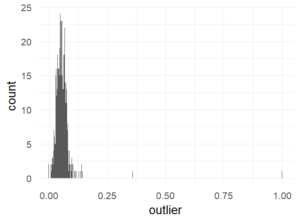Percentage of trials marked as outliers. This is well in the recommended range of 10% (Ratcliff).

### References

Bolota, D.A., Yap, M.J., Cortese, M.J., Hutchison, K.A., Kessler, B., Loftis, B., Neely, J.H., Nelson, D.L., Simpson, G.B., & Treiman, R. (2007). The English Lexicon Project. Behavior Research Methods, 39, 445-459. – http://elexicon.wustl.edu/about.asp

library(ggplot2)
library(dplyr)
theme_set(theme_minimal(20))

d$Sub_ID = factor(d$Sub_ID)
d$D_RT = as.integer(d$D_RT)

d = d%>%group_by(Sub_ID)%>%mutate(outlier = abs(D_RT-median(D_RT))>3*mad(D_RT,na.rm = T))
d$outlier[d$D_RT<1] = TRUE
d$outlier[is.na(d$D_RT)] = TRUE

# outlier plot
ggplot(d%>%group_by(Sub_ID)%>%summarise(outlier=mean(outlier)),aes(x=outlier))+geom_histogram(binwidth = 0.001)

fft_on_hist = function (inp){
maxT = 4
minT = 0
fs = 1000

h = hist(inp$D_RT,breaks = 1000*seq(minT,maxT,1/fs),plot = F) h = h$counts;

# I tried to use density estimates instead of histograms, but it was difficult
#h = density(inp$D_RT,from = minT,to=4000,n=4000) #h = h$y
f = fft(h)
f = abs(f[seq(1,length(f)/2)])

return(data.frame(power = f, freq = seq(0,fs/2-1/maxT,1/maxT)))
}

d_power = d%>%subset(outlier==F)%>%group_by(Sub_ID)%>%do(fft_on_hist(.))

ggplot(d_power,aes(x=freq,y=(power),group=Sub_ID))+geom_path(alpha=0.01)
ggplot(d_power,aes(x=freq,y=log10(power)))+geom_path(alpha=0.01)

ggplot(d_power%>%group_by(freq)%>%summarise(power=mean(power)),aes(x=freq,y=(power)))+geom_point()+stat_smooth(method='loess',span=0.1,se=F,size=2)+xlim(c(10,250))+ ylim(c(47,53))



## Interaction and Effect/Sum Coding

Some time ago I wrote a blog post on dummy & effect coding. I made some new plots to visualize why the interaction in sum/effect is coded as it is.

Let’s take a typical 2×2 design. We have two 2-level factors $A$ and $B$ and we also allow for an interaction.
$$y \sim A + B + A:B$$

We code A with -1 / 1 and B with -1 / 1 (depending on the level e.g. On=1, Off=-1)

The interaction is coded as the multiplication of A and B: $A * B$. Therefore if $A$ and $B$ are both in the same level (both “off” or both “on”) we get a $+1$, else a $-1$.

Side remark: This is different in dummy/reference coding, where the interaction only codes what is extra if both A & B are “on” (turns out that the magnitude of the interaction is just double – but this is a story for another time).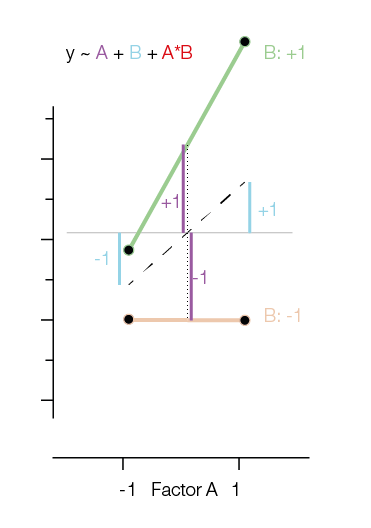In the first figure I added the main effects of $A$ and $B$ as Blue and Purple lines. The main effects in reference coding are relative to the means of the group means.

In order to model the original data points, one needs to add the main effects and the interaction together: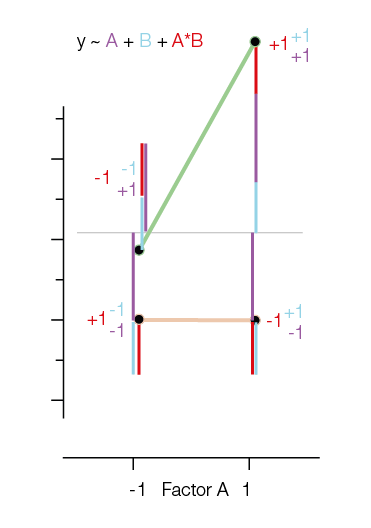Notice that the way we have to add the interactions and main effects is exactly the multiplication I introduced earlier. That is, if we need to take -1 for $A$ and +1 for $B$, you bet we will need -1 for $A:B$.

One way that I like to think about the interaction in effect coding is to think “What would be my prediction if there would be no interaction?”.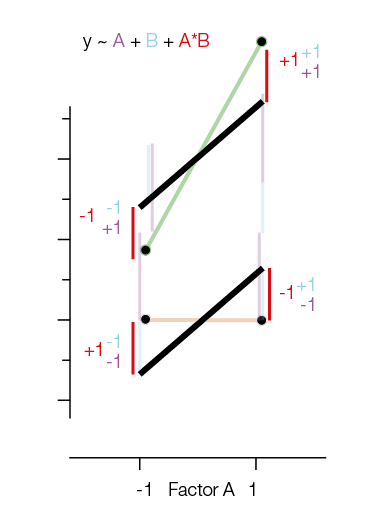“What if there would be a model without interaction” is marked in black (it’s only using the main effects!). Note that the two black lines are parallel. Adding the red interaction-lines again helps us to move to the original datapoints.

## Trains and EEG

I had this blog post as a draft for nearly a year now… and finally here it is: In my last lab we moved buildings. Good scientists we are we did measurements to see if the system works as intended…

### The EEG Systems

• An active 64 electrode system from BrainProducts called ActiCap
• A passive 128 electrode system from ANT, a tmsi REFA-8 with actively shielded cables
• A passive 128 electrode system from ANT, eegosport with actively shielded cables

Disclaimer: All data shown here are from different subjects. Their individual alpha-peaks etc. will differ, we also switched methods a bit

### ActiCap

We measured 2 subjects with the 64 electrode system. When we checked the frequency spectrum we were very worried.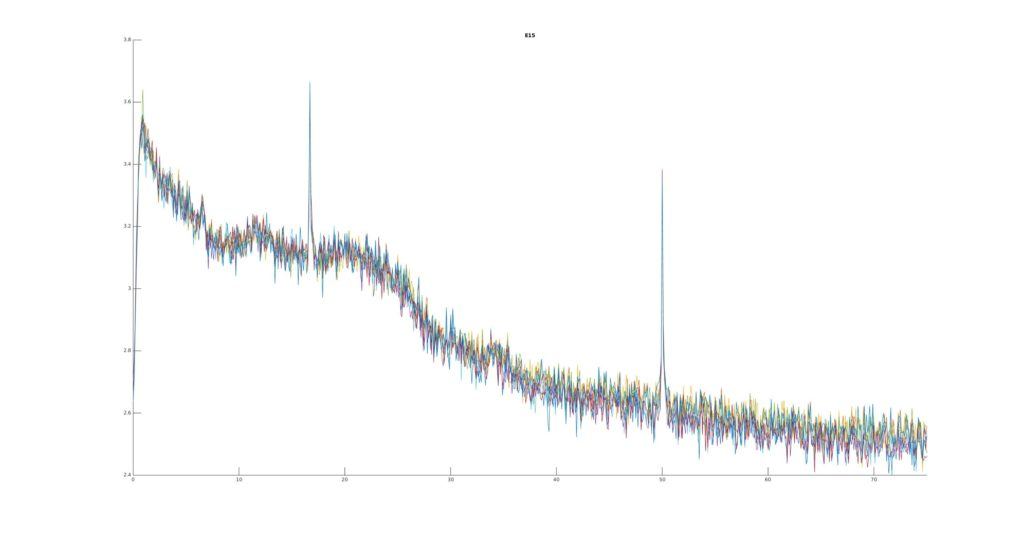Besides the typical 50Hz artefact that we know, one can clearly see a $16 \frac{2}{3}$ Hz peak in the beta-band. The artefact is very strong and variable over time.

This can’t be right? So we recorded another subject, just to be sure. We also switched analysis methods a bit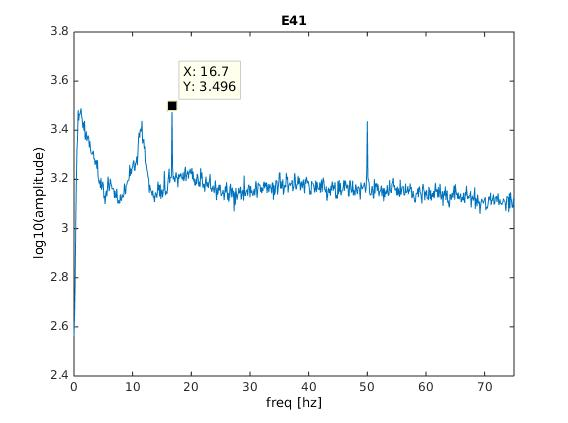Same thing!

Side remark: The ‘activeShield’ button did not improve the shielding (plots not shown, the support told us that the activeShield function is more thought for passive electrode systems, not our system).

### ANT/TMSI REFA-8

#### Single Amplifier (64 Electrodes)

We then measured a single subject with the ANT system with a single amplifier.

All looks fine here for now… On the second plot, we introduce the signal-to-noise (SNR) index, which is simply the current frequency-bin divided by neighbouring bins (the average of $n_{-12}$:$n_{-2}$ & $n_{+2}$:$n_{+12}$). This will enhance peaks in the spectrum. As we can see, we do not see any peaks anywhere, all is fine with 64 electrodes.

#### Two Amplifiers (128 Electrodes)

At first we thought, cool! ANT with active shielded cables is perfect, we will just not record with the brainproducts system. But ANT/tmsi allows to “chain” two amplifiers together to effectively get 127 electrodes. That is, the amplifiers share a ground electrode and a single electrode is bridged, so that the data can be referenced to a amplifier-common electrode. When we did this the following picture emerges: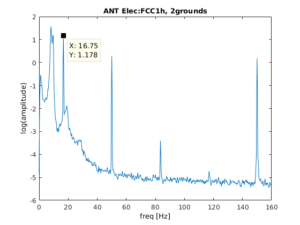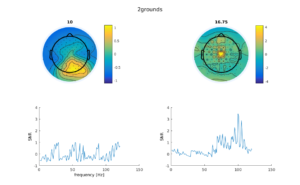The 16 Hz is back! :(. The electrode depicted in the spectrum plot is from the second amplifier. In the lower right panel we can see, especially in the second plot, that the second amplifier has a lot of 16Hz artefact but not so much in the first amplifier.

#### ANT eegosport

We much later received an ANT eegosport for testing purposes. Unfortunately for us (and ANT because we did not upgrade ;)), the artefact was also present with comparable strength. Here an interesting new phenomenon arised: when flipping the amplifiers upside down, the artefact switched from channel 1-32 to 33-64 or vice versa.

### Typical artefact behavior

The artefact was non-stationairy over time (sometimes its there, sometimes not, stronger, weaker …). Most often observed in the second amplifier, sometimes in the first. The artefact also was stronger (relatively) around the reference electrode. It does not depend on exact placement in room. We tried a lot of factors, at some point even wrapping things in foil – it did help a bit!

### What the hell is going on?

Turns out, the new building is quite close to train tracks. In Northern Germany most trains are powered electrically using overhead lines. Germany uses $16 \frac{2}{3}$ Hz to power these lines. So sometimes when a train comes along we have an artefact. Because we did not want to time our experiments to the train schedule (we a) thought about it, but the DeutscheBahn does not give away information when trains are coming and b) the artefact is not _always_ strong when a train comes, but only sometimes, also not train dependent – but who knows…)

### What can we do against it?

It took us the most part of the year (with lots of discussion with ANT & TMSI) to figure out, where exactly the artefact is induced into the signal.

### Our solution

• Keep the cables as close together as possible, all in one orientation. Because the ANT REFA system allows the ~10cm cable to go into various direction, the common-noise which influences these channels can be slightly different. If the electrical field / artefact is strong enough, this slight differences will show up in your data. Thus always keep all cable very similar. We now “comb” the connector cables
• Keep the cables parallel to the field-lines of the electrical field. I.e. don’t put your cables parallel to the elongated electrical source, this will induce stronger effects. We now lead the cables to the top, so inline with the electric field
• If you use a cascaded system, the reference-electrode that links the two systems, has to follow both strings of electrodes. I.e. you have to guide it along the connector. If you fail to do so, there will be stronger artefact in one than in the other amplifier (depending on how the cable lays relative to the artefact electrical field). We now take care of this.

Combining all these measures helped us enourmously to reduce the artefact in a stationairy setting. For mobile recordings this will be tougher.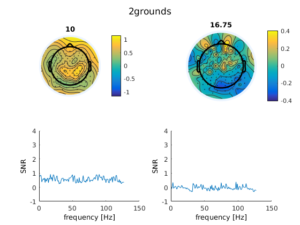### Debugging Lessons Learned

It took us a long time to figure out how to measure the artefact reliably and how to manipulate the system in order to study the artefact. Here are some lessons learned:

• A waterbucket+salt (=> a head without needing a human) is your friend. BUT: We tried this earlier and failed because the system was DC-maxing out (no signal in range). This was because we put in the whole ground electrode (which has a zink-connector) and not only the tip (AgCl). This gives very large DC offsets due to the zink contact and you cannot measure.
• Making turn around times super fast. This allows for more tests and easier replication of supposedly found effects. A online/live-script that shows us the current 16Hz power was very helpful here but took quite some programming to get smoothly.
• You need to show a lot of own initiative, support can help you, but not save you. This problem was very specific for us, a general solution did not exist
• If there is a non-stationairy noise source, it is very helpful to monitor the artefact with one amplifier while modifying things with the other amplifier. Only like that can you be sure that the artefact occured / your modification actually changed something (and you did not just measured in a low-artefact regime). An interesting phenomenon we saw, was that on one day after ~19.00 the artefact vanishes. We are not sure why this is the case, because trains were still running. The trains passing by do not produce a reliable effect in itself. We think this is because the power to the grid is turned on whenever a train is on the whole section, with a section being multiple kilometers long and possibly reaching into the trainstation (but we do not know this before).

### Methods

We detrended or highpass filtered the data at 1Hz. Welchs estimate does not like DC-drifts. We generally either bandpass filter the data, or use the pwelch algorithm. We also employed the TMSI matlab-toolbox and programmed a live-script to monitor the 16Hz activity while manipulating the EEG. We also used some normalization to compare the signal to its frequency-bin neighbours. This allows for relative noise effects that show up when re-referencing to a single electrode.

I uploaded the tmsi EEG live-script on github

## Thesis Art: Lisa-Marie Vortmann

I was a supervisor for Lisa-Marie Vortmanns’s Master’s Thesis.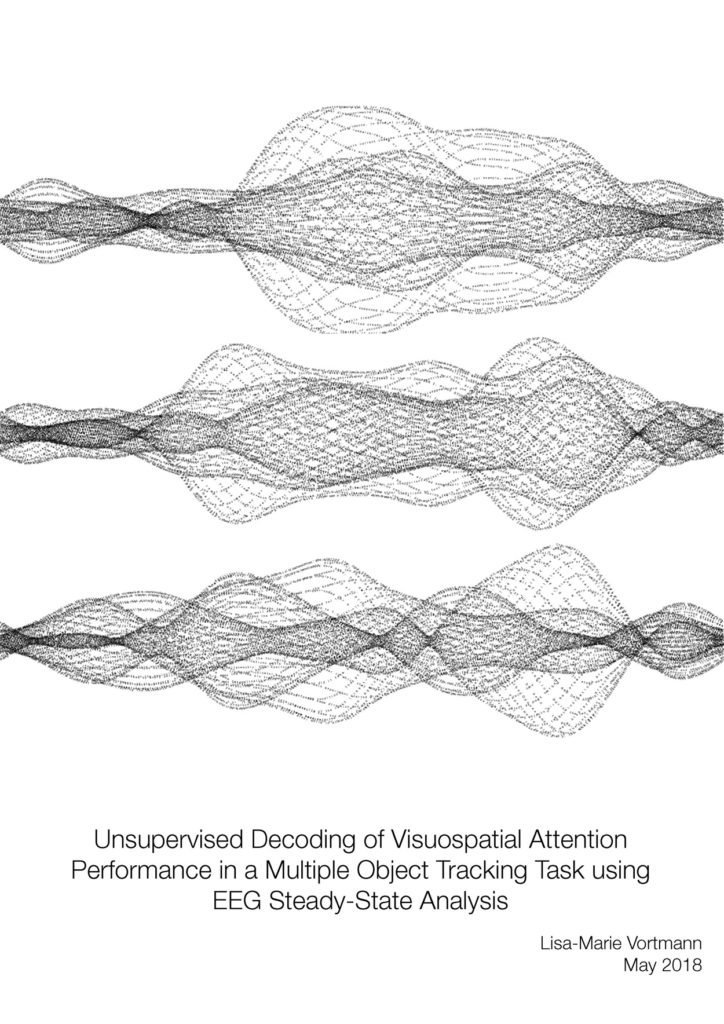In her project, we tried to decode from brain activity* which objects on a screen a subject is currently tracking with their mind. To do this, we “tagged” the to-be-tracked objects and distractor with different frequencies, so that we can trace them in the EEG. What you see here is the SSVEP response over time for three trials per row. At the beginning and end, the response is small because the object was not tracked, only in the middle part one can see that the response is much larger, the object was tracked. The plot is “written” by the first 10.000 letters of Lisa’s Thesis.

The idea of “thesis art” is to inspire discussion with persons who do not have an academic background or work in a different field. The thesis is hidden in the drawer, but the poster is out there at the wall for everyone to see. You can find all past thesis art pieces here

* This is actually Lisa’s own brain activity

I showed a student how I would improve a plot. The result is I think typical for what I would design. The details change for each paper and I do not have a specific “style” I wanted to emulate. I usually enjoy the before & after of other graphics, so here is mine: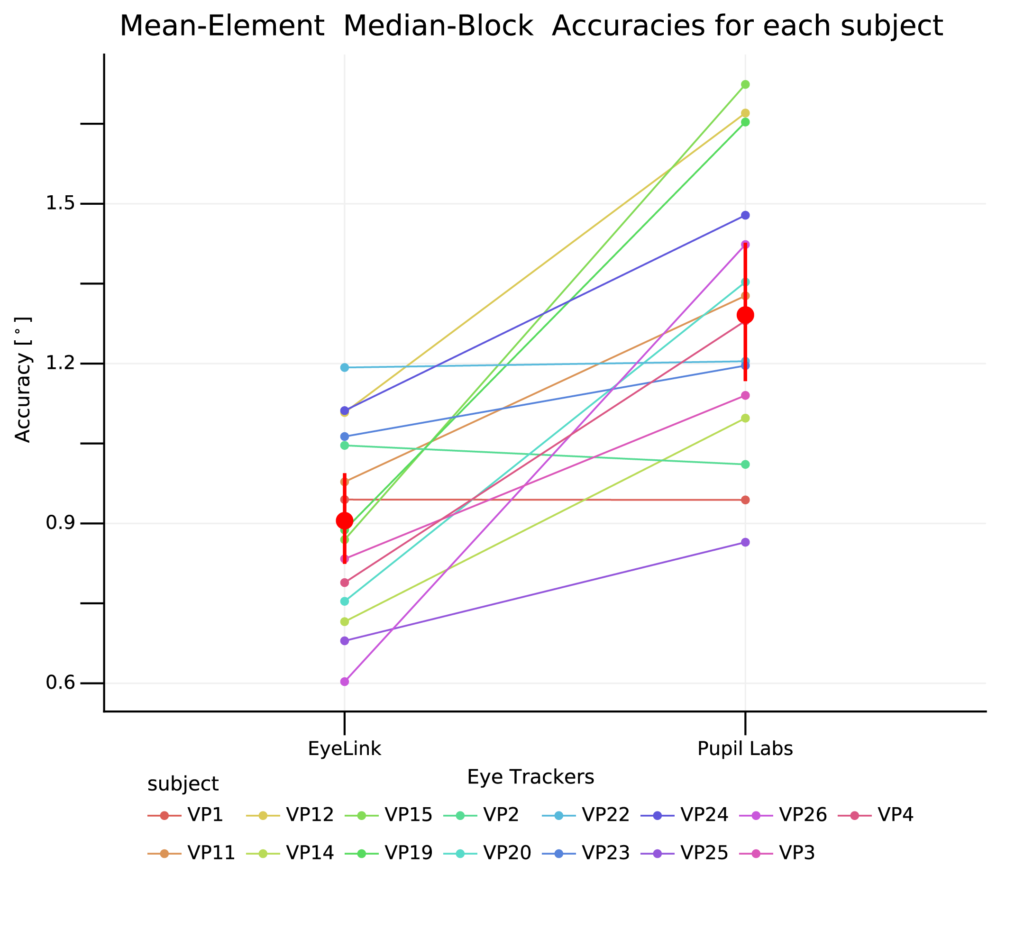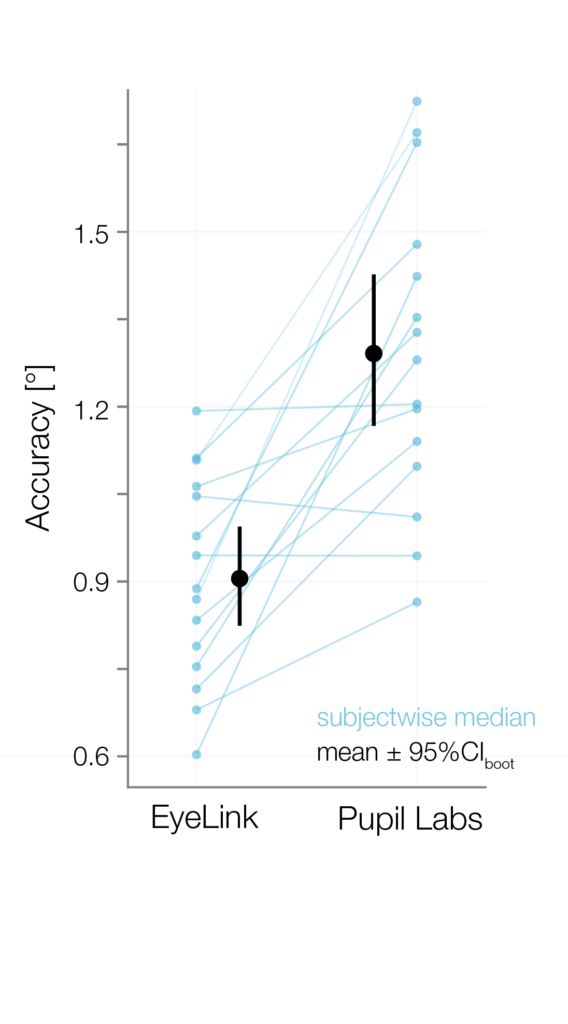PS: The data shown in the plots are preliminary

## Thesis Art: Maria Sokotushchenko

I was a supervisor for Maria Sokotushchenko’s Master’s Thesis.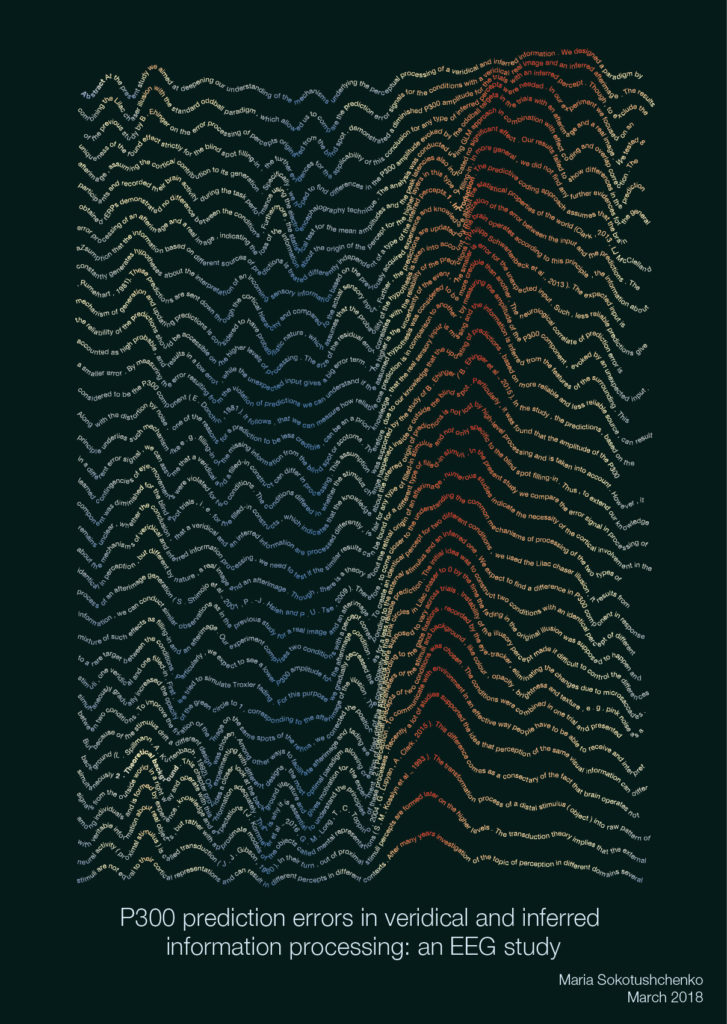In her thesis-art, I artistically visualized the brain’s surprise response to a unexpected stimulus change. This response is sorted by how fast subjects responded (late on top, fast in the bottom)

The idea of “thesis art” is to inspire discussion with persons who do not have an academic background or work in a different field. The thesis is hidden in the drawer, but the poster is out there at the wall for everyone to see. You can find all past thesis art pieces here

2 of 5
12345